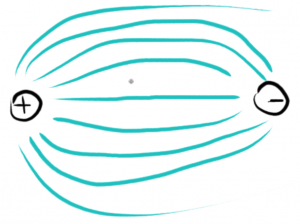# Electric Fields – (Part 2 of 2)

Part 1 of Electric Fields is here: Electric Fields (Part 1 of 2)

• Fe = K | q1 q2 | / r2• The further away, the weaker the electric fields force becomes.
• Eventually, the force won’t be attracted enough, and it won’t reach the opposite charge:## Electrical Potential Energy

Remember that `Pe = m . g . h`

Pe = Gravitational potential energy

m is the mass, g is gravity, and h is the height

• Work = Force of Gravity (Fg) × Height (h)
• W = Fg × h

Electrical potential energy is very similar to gravitational potential energy.

For example:

• Moving A to position B
• Electric field strength: E = 5 N/c
• Field force = Ff = 5 N/c × 2 c = 10 N
• Distance between A, and B is 3 meters

↑      . A (+2 c)      ↑      ↑     ↑    ↑      ↑

↑     . B (+ 2c)  ↑      ↑     ↑       ↑      ↑

_____________________________

< ++++++++++++++++++++++++++ > (this is an infinite plate)

#### Q. How much work does it take to move a +2 c charge, down 3 meters?

The plate is positively charge – as is the ‘floating’ charge, so these forces will repel each other.

• Total upward force:
• Ff = 5 N/c × 2 c = 10 N
• Work = 10 N  × 3 m
• Work = 30 N/m = 30 J of energy

The electrical potential energy: Pe (Prelative to Pb) = 30 J

This is NOT electric potential – this is electrical potential energy

Electric potential = work / charge = 30 J / 2 c = 6 J/c = 6 V (Volts, Voltage)